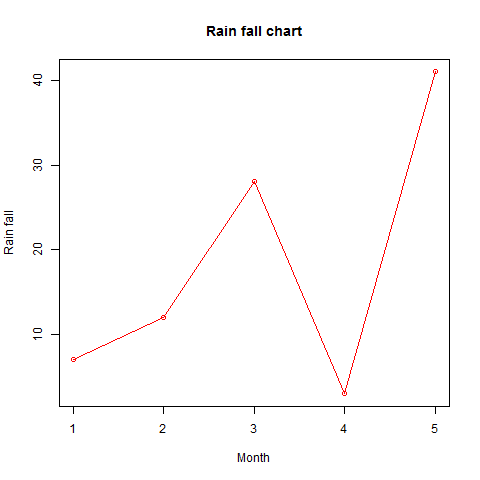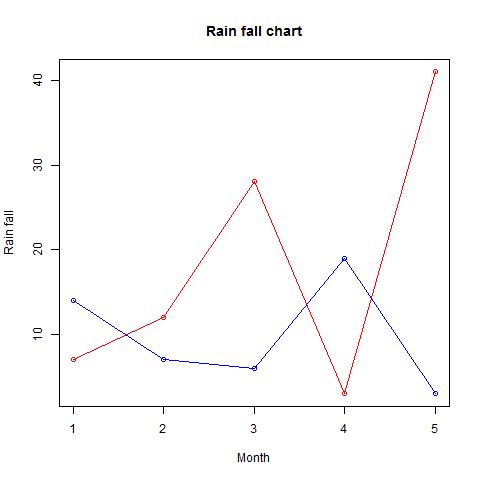# R语言 折线图

R语言中的plot()函数用于创建折线图。

## 语法

```plot(v,type,col,xlab,ylab)
```

• v是包含数值的向量。
• 类型采用值“p”仅绘制点，“l”仅绘制线和“o”绘制点和线。
• xlab是x轴的标签。
• ylab是y轴的标签。
• main是图表的标题。
• col用于给点和线的颜色。

## 例

```# Create the data for the chart.
v <- c(7,12,28,3,41)

# Give the chart file a name.
png(file = "line_chart.jpg")

# Plot the bar chart.
plot(v,type = "o")

# Save the file.
dev.off()
```## 例

```# Create the data for the chart.
v <- c(7,12,28,3,41)

# Give the chart file a name.
png(file = "line_chart_label_colored.jpg")

# Plot the bar chart.
plot(v,type = "o", col = "red", xlab = "Month", ylab = "Rain fall",
main = "Rain fall chart")

# Save the file.
dev.off()
```## 多线型折线图

```# Create the data for the chart.
v <- c(7,12,28,3,41)
t <- c(14,7,6,19,3)

# Give the chart file a name.
png(file = "line_chart_2_lines.jpg")

# Plot the bar chart.
plot(v,type = "o",col = "red", xlab = "Month", ylab = "Rain fall",
main = "Rain fall chart")

lines(t, type = "o", col = "blue")

# Save the file.
dev.off()
```## 【阿里云】棒款特惠精选

#### 云服务器低至8.1元/月 - 开始搭建你的博客吧